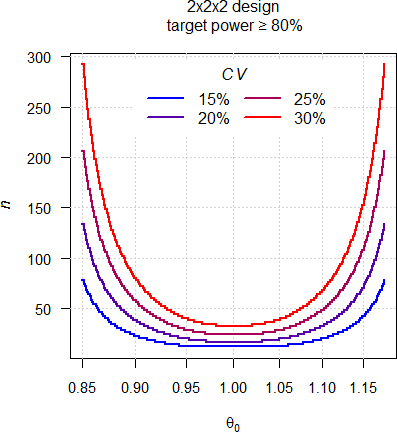## R-Code for Power in 2×2 Cross-over [🇷 for BE/BA]

Hi Brus,

❝ I am trying to adapt your example of power calculation with plot replesentation, but for the calculation of the sample size. My objetive is to plot a graphicala representation with ratio in X axis and sample size in Y axis and setting the CV and power as a fixed value.

I suggest this goody first. May sound picky but we can’t get the exact desired power, only one which is at least that high.*

Try this one:

library(PowerTOST) design <- "2x2x2" # any in known.designs() target <- 0.80    # target (desired) power x.min  <- 0.85    # minimum T/R-ratio (> 0.8) x.res  <- 250     # 'resolution' of the x-axis y.min  <- 0.15    # minimum CV y.max  <- 0.30    # maximum CV y.res  <- 0.05    # step size of CVs # T/R-ratios equally spaced in log-scale theta0 <- exp(seq(log(x.min), log(1 / x.min), length.out = x.res)) CV     <- seq(y.min, y.max, y.res) res    <- data.frame(CV = rep(CV, each = length(theta0)),                      target = target, theta0 = theta0,                      power = NA_real_, n = NA_integer_) for (j in 1:nrow(res)) {   tmp <- sampleN.TOST(CV = res$CV[j], theta0 = res$theta0[j],                       targetpower = target, design = design,                       print = FALSE) # no output to the console   if (tmp[["Sample size"]] >= 12) {  # full throttle     res[j, 4:5]  <- tmp[8:7]   } else {     res$n[j] <- 12 # acc. to the guidelines res$power[j] <- power.TOST(CV = res$CV[j], theta0 = res$theta0[j],                                design = design, n = res$n[j]) } } # theta0 on log-axis to demonstrate the symmetry plot(res$theta0, res$n, type = "n", log = "x", las = 1, ylim = c(12, max(res$n)), font.main = 1,      main = paste(design, "design\n",                   sprintf("target power \u2265 %.f%%", 100 * target)),      xlab = expression(theta), ylab = expression(italic(n))) grid() clr <- colorRampPalette(c("blue", "red"))(length(CV)) for (j in seq_along(CV)) {   lines(res$theta0[res$CV == CV[j]],         res$n[res$CV == CV[j]], lwd = 2, type = "s", col = clr[j],         lend = 2, ljoin = 1) } legend("top", bg = "white", inset = 0.02, box.lty = 0,        legend = sprintf("%.f%%", 100 * CV),        title = expression(italic(CV)), lwd = 2, col = clr,        seg.len = 2.5, ncol = 2)The sample size is a staircase function and due to the symmetry in $$\small{\log_{e}}$$-scale we require the same sample size for any given $$\small{\theta_0}$$ and $$1/\small{\theta_0}$$ (see also there).

• OK, if we would be able to dose fractional subjects, then yes. I can’t. Amazingly some software packages report sumfink like $$\small{n=23.45}$$ and leave the rounding up to the user. Crazy.

Diving deeper into the matter: Power is a hypersurface depending on $$\small{\theta_0}$$, $$\small{n}$$, and $$\small{CV}$$. We can only show spatial projections at certain $$\small{CV}\text{-}$$values (the panels) with $$\small{\log_{e}\theta_0}$$ (x-axis), $$\small{n}$$ (y-axis), and power (z-axis).
$$\small{n=12-292}$$ and $$\small{\theta_0=0.80-1.25}$$ to demonstrate that at the limits of the acceptance range power (which is there the Type I Error) is ≤0.05.The intersections with the horizontal plane (the target power) show the U-shaped dependency of $$\small{n}$$ on $$\small{\theta_0}$$ as in the plot above. The slices at certain $$\small{\theta_0}$$- and $$\small{n}$$-values give the plots like in the very old post above.

Don’t try to imagine four-dimensional objects. Even a simple hypercube (tesseract) may fry you brain.CC0 2007 Jason Hise @ Wikimedia Commons

Dif-tor heh smusma 🖖🏼 Довге життя Україна!Helmut SchützThe quality of responses received is directly proportional to the quality of the question asked. 🚮
Science QuotesIng. Helmut Schütz